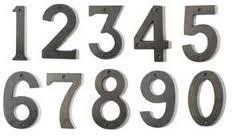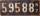# Two numbers

Find two numbers whose difference and ratio is 2.

Correct result:

a =  4
b =  2

#### Solution:

$a=2+\frac{2}{2-1}=4$
$b=\frac{2}{2-1}=2$Our examples were largely sent or created by pupils and students themselves. Therefore, we would be pleased if you could send us any errors you found, spelling mistakes, or rephasing the example. Thank you!

Please write to us with your comment on the math problem or ask something. Thank you for helping each other - students, teachers, parents, and problem authors.Tips to related online calculators
Check out our ratio calculator.
Do you have a system of equations and looking for calculator system of linear equations?

## Next similar math problems:

• RatioIncrease in the ratio 20:4 number 18.5.
• Two numbersDetermine the numbers x and y so x + y = 8 is truth and the numbers are in the ratio of 4: 5.
• Arble bagA marble bag sold by Rachel's Marble Company contains 5 orange marbles for every 6 green marbles. If a bag has 35 orange marbles, how many green marbles does it contain?
• DonutsFind how many donuts each student will receive if you share 126 donuts in a ratio of 1:5:8
• Boys and girls 2The ratio of boys to girls in math club is 4:3 . After 8 more girls joined the Club, the ratio become 1:1. How many members are there in the club now?
• WeightsMarry and John together weighing 49 kg. Their weights are in ratio 1:6. Determine their weights.If Petra read 10 pages per day, she would read the book two days earlier than she read 6 pages a day. How many pages does a book have?
• 1.5 divided1.5 divided by 1 = w divided by 4
• Two trainsThere were 159 freight wagons on the railway station creating 2 trains. One had 15 more wagons than the other. How many wagons did each train have?
• Spain vs USASpain lost to the US by 4 goals. In the match total fell 10 goals. How many goals gave the Spain and how the United States?
• Boys and girlsThere are 48 children in the sports club, boys are 10 more than girls. How many girls go to the club?
• The pigletThe weight of the piglet grew regularly by five kilograms for four months. Determine the proportion by weight of the piglet in each month, if the first weighed 35 kg.
• Linear systemSolve this linear system (two linear equations with two unknowns): x+y =36 19x+22y=720
• HostelStudents are accommodated in 22 rooms. Rooms were 4 and 6 bed. How many rooms in which type occupied 106 children there?
• Simple equationsSolve system of equations: 5x+3y=5 5x+7y=25
• Grandfather and grandmotherThe old mother is 5 years younger than the old father. Together they are 153 years old. How many years has each of them?
• Waiting roomIn the waiting room are people and flies. Together they have 15 heads and 50 legs (fly has 6 legs). How many people and flies are in the waiting room?